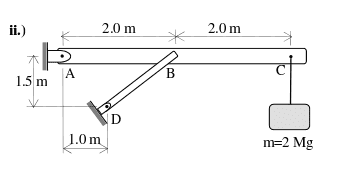# Is this mechanics question possible to solve?

## Homework Statement

Determine all support reactions for the system shown. Indicate the resultant magnitudes and direction angles of the unknowns. Neglect thickness and self-weights of the members.## Homework Equations

Equilibrium equations of a rigid body:
1. The moment about any point is zero: M_o = 0
2. The sum of the x components is zero: F_x = 0
3. The sum of the y components is zero: F_y = 0

## The Attempt at a Solution

First I drew a FBD to determine...
1. point A has two force components, A_x (-x), A_y (+y).
2. point D is a two force member, thus it has two components: Dcos(theta)(+x), Dsin(theta)(+y)
3. point C force is (2000kg*9.8m/s^2)(-y)

I then plugged this info to three equations to find:

1. D = 7800 Nm / ( sin(theta)*m + cos(theta)*1.5m )
2. D = A_x/cos(theta)
3. A_y = 19600 N - Dsin(theta)

I don't have enough information to solve this problem. So I don't know what to do.

Is it possible to solve this problem? What should I do?

Orodruin
Staff Emeritus
Homework Helper
Gold Member
I suggest you try to draw free body diagrams for all members, this will allow you to dissect the problem into its components pretty fast. Typically, if you get a construction that is rigid, there will be enough equations available to determine the forces in equilibrium.

•x86
Yes. I drew a FBD for ABC, DB, and for both of these structures combined. However, I couldn't find anything besides what I listed in my original question. I'm not really sure if I'm just not seeing something, or if the question just can't be solved with the presented information.

SteamKing
Staff Emeritus
Homework Helper
Yes. I drew a FBD for ABC, DB, and for both of these structures combined. However, I couldn't find anything besides what I listed in my original question. I'm not really sure if I'm just not seeing something, or if the question just can't be solved with the presented information.

Try analyzing just the bar ABC first. Write the equations of equilibrium for this bar alone. Once it is solved, you can come back and look at bar DB.
Try analyzing the vertical forces first; the horizontal forces are trivial.

•x86
Try analyzing just the bar ABC first. Write the equations of equilibrium for this bar alone. Once it is solved, you can come back and look at bar DB.
Try analyzing the vertical forces first; the horizontal forces are trivial.

I think I did that, unless you mean to ignore point B, which I don't think I can do.

My FBD for rod ABC is like this:
at A it has two forces, one up, one to the left
at B it has a force up, a force to the right
at C it as a force down

My FBD for rob DB is like this:
At D it has a force to the right and up
at B it has a force to the left and down.

I still get what I got in the first post

Orodruin
Staff Emeritus
Homework Helper
Gold Member
You have six equations (two force equilibria and one momentum equilibrium for each part). Since you have six unknowns (two components for each of the three forces - I am here counting the forces at B only once as they are a force pair equal magnitude and opposite direction according to Newton III), you should be able to solve this. I would suggest doing momentum equilibrium around B for both FBDs.

•x86
You have six equations (two force equilibria and one momentum equilibrium for each part). Since you have six unknowns (two components for each of the three forces - I am here counting the forces at B only once as they are a force pair equal magnitude and opposite direction according to Newton III), you should be able to solve this. I would suggest doing momentum equilibrium around B for both FBDs.

EDIT: Ah okay. I understand now. I was having issues with my FBD but understand why I was wrong now. Thank you.

Orodruin
Staff Emeritus